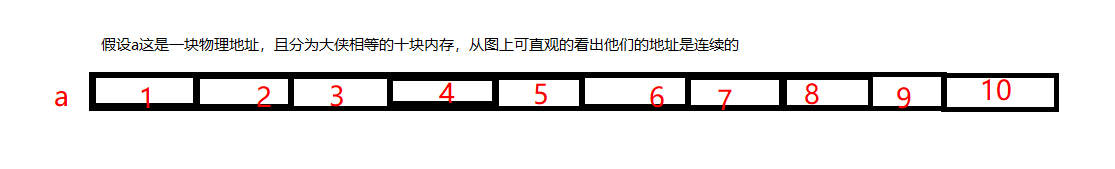## 定义：同一种类型数据的集合

`//eg#include<iostream>using namespace std;​void main(){ int a;    //等效于 int a; int num; //一般用于定于多个，此处就表示，定义10个int类型的数据         //注意数组是从零开始计数的}`

`//eg#include<iostream>using namespace std;​void main(){    int a;   //假如你现在需要十个正整型数据 先赋值再求和    int sun = 0;  //定义sum的初始值为0    for(int i = 0; i < 10; ++i)   {        a[i] = i;   }    for(int j = 0; j < 10; ++j)   {        sum += a[j];   } cout << sum << endl;}`

`#include<iostream>using namespace std;void main(){ int a = { 0, 1, 2, 3, 4, 5, 6, 7, 8, 9 };  //我们可以先去打印a 与 a之间的内存差看看效果 cout << &a <<" "<< &a  << endl;     //& 在这里是取地址符 cin.get();}`## 数组的初始化

`#include<iostream>using namespace std;int main(){ int a = {0}; //这种方式将默认是个元素全部为零    int b = {0,1,2,3,4,5,6,7,8,9};//一一对应的方式。    //也可以在后续的过程中给出自己的操作    return 0;}`

#### 注意！

C/C++一维数组与指针 C/C++ 一维数组与指针 C++指针与一维数组 【C++】 引入一维数组的元素 学习C++ -> 一维数组